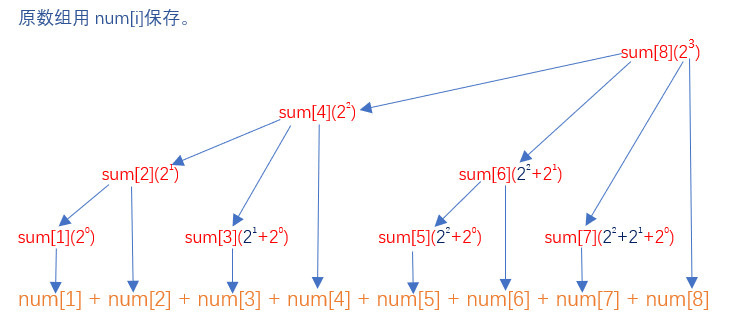# 树状数组的使用

### 1.树状数组的建立：

……  ……  ……  ……  ……

//lowbit操作
int lowbit(int x) {
return x & (-x);
}

0001     ：num

0010：     ：num+num

0011     ：num

0100：     ：num+num+num+num

0101     ：num

0110：     ：num+num

0111     ：num

1000：     ：num+num+num+num+num+num+num+num1.树的深度为logn；

2.第x个结点的父结点为x+lowbit(x);

3.第x个结点的子结点个数为lowbit(x);

for(int i=1; i<=n; ++i){
cin >> x;
update(i, x);
}

const int N = 100005;
long long num[N], sum[N];
int n;//数据规模

//建立树状数组;
void build(){
for(int i=1, x; i<=n; ++i){
sum[i] += num[i];
x = i + lowbit(i);
if(x <= n)
sum[x] += sum[i];
}
}


### 2.区间询问：

//求num到num[x]的和
long long solve(int x) {
long long  ans = 0;
for(int i=x; i>0; i-=lowbit(i))
ans += sum[i];
return ans;
}

### 3.单点修改：

//单点修改，x位置+y
void update(int x, int y) {
for(int i=x; i<=n; i+=lowbit(i))
sum[i] += y;
}

### 4.与差分数组结合后实现区间修改：

### 5.二维树状数组：

//将(x，y)的值+z
void update(int x, int y, int z) {
for(int i=x; i<=n; i+=lowbit(i))
for(int j=y; j<=m; j+=lowbit(j))
sum[i][j]+=z;
}
//从（1，1）到（x，y）的和
long long solve(int x, int y) {
long long ans = 0;
for(int i=x; i>0; i-=lowbit(i))
for(int j=y; j>0; j-=lowbit(j))
ans += sum[i][j];
return ans;
}

# 例题及补充：

template<typename type>
void read(type &num){
int sign=1;
long long res=0;
char ch=getchar();
while (!isdigit(ch))
sign=ch=='-'? 0:1,ch=getchar();
while (isdigit(ch))
res=res*10+(ch^48),ch=getchar();
num=sign? res:-res;
}

3.A Tiny Problem with intergers（https://ac.nowcoder.com/acm/contest/1032/B

其余的东西太占篇幅了，干脆不写了。。。
for(int i = 1; i <= n; ++i){
cin >> num[i];
update(i,num[i]-num[i-1]); //在输入的时候就构造差分数组
}
//输出时就不用加上原数组的值
cout<<solve(a)<<"\n";


同样占篇幅，不写，就是这么任性。。。
for(int i = 1; i <= n; ++i)
cin >> num[i];      //输入时未构造差分数组
//输出时要加上原数组的值
cout<<num[a]+solve(a)<<"\n";


4.A Simple Problem with Integers（https://ac.nowcoder.com/acm/contest/1032/C

04-01116108-211110
08-063963
05-151万+
09-142154
10-041621
03-1487
12-02516
05-16280
06-241693
12-0542
10-1765
08-09158
08-0455
10-06637
03-20234
08-02365
©️2020 CSDN 皮肤主题: 像素格子 设计师:CSDN官方博客